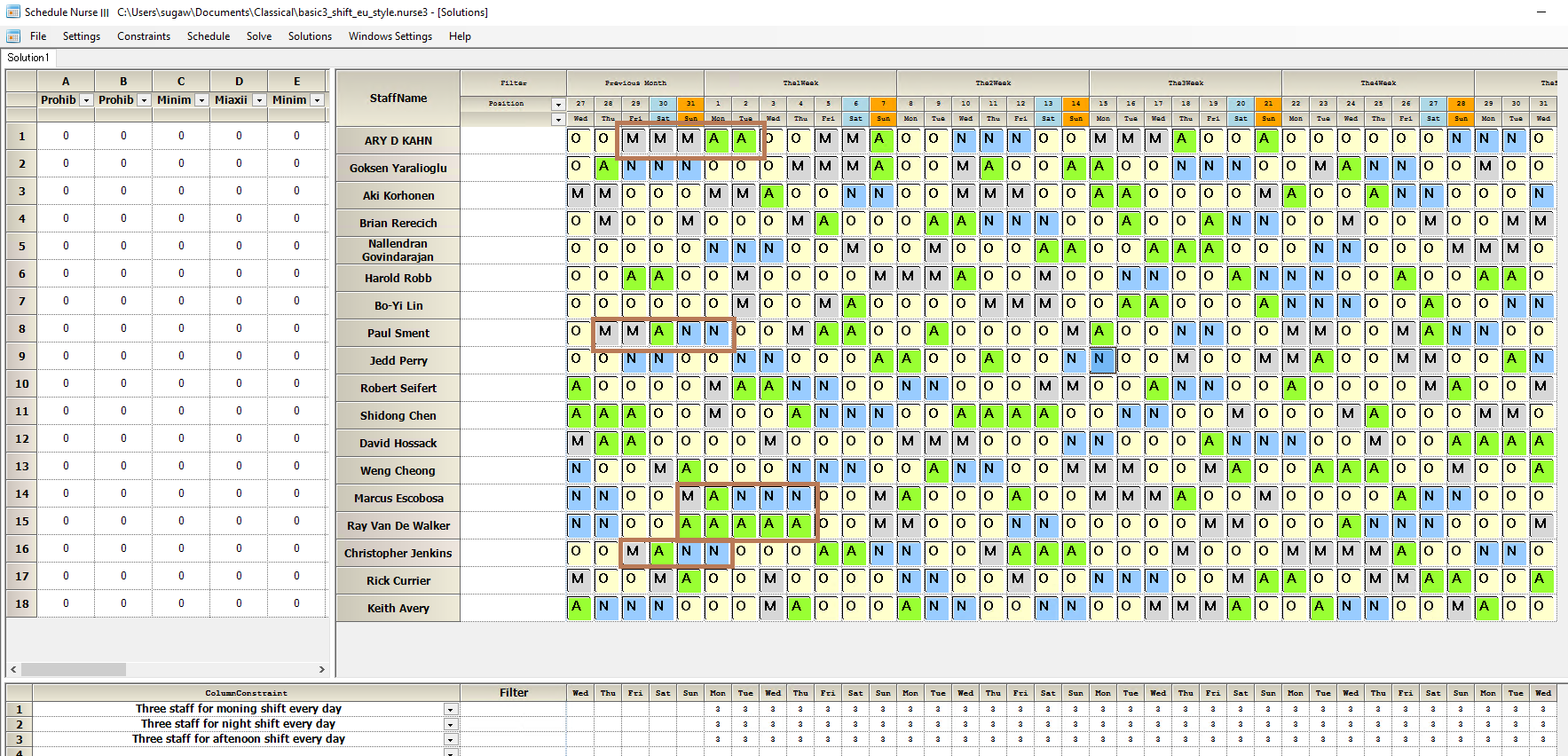# Knowledge Base

2022-05-25

## What is hard constraint?

In an optimization model, a hard constraint is a constraint that must be satisfied by any feasible solution to the model. Remember, no solution breaks hard constraints. At the same time, you must write Hard Constraints that do not violate any rules by themselves. For example, consider following hard constraints.
１）Σx(i)>=3
２）Σx(i)<=2
It is clear no solution satisfies both 1) and 2). You may think it is silly to write such constraints. Indeed, it is a clear case that you can detect easily in a simple problem. However, there are so many constraints in actual nurse scheduling problems that implicitly violate the rule if you write rules only with Hard Constraints.

On the other hand, there are soft constraints. You can violate soft constraint rules. However, a weighted cost will incur if you violate the rules. Since the system tries to minimize the total cost, it tries to follow the soft constraints as much as possible, especially on more significant weight constraints..

So your question may arise why not just make everything with soft constraints? It is unreasonable that there is no solution.

The answer is a good system has an appropriate mix of hard and soft constraints.

Technically speaking, soft constraints do not reduce the search space, but they increase the solution space significantly. As an optimizing solution designer, this is not something I am happy about. On the other hand, a hard constraint can dramatically reduce the search space. From this alone, we are happy, but at the same time, it also reduces the solution space. Therefore, it also increases the possibility of not finding a solution.

Even with supercomputers, only a few random binary variables are known to be solvable, about 70 variables by enumerating all combinations. However, thanks to the Hard Constraints, we can sometimes solve the nurse scheduling problem on a scale more significant than the Search Space 1googol=1e100.

## What is a constraint?

A constraint is a condition of an optimization problem that the solution must satisfy. The set of candidate solutions that satisfy all constraints is called the feasible set. The nurse scheduling problem consists of many constraints. It is necessary to meet all Constraints to get a feasible solution. In other words, if a AND set of the constraints becomes to be an empty set, then there is no solution. It is easy if the Constraints are two or three to use a Venn diagram, but note the number of Constraints is hundreds of thousands in the nurse scheduling problem if we look into it in detail.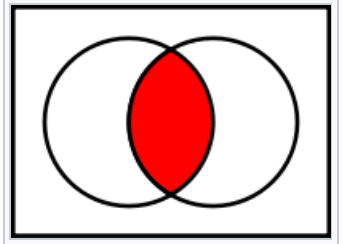This situation is similar to piling up the building blocks of Constraint so that they do not fall over.When we have piled all the building blocks up, the solution exists, but if it falls over, there is no solution due to an empty set in the middle.## How do you write a soft constraint?

I explain it using a problem of Second International Nurse Rostering Competition (INRC-II).
There are four types of soft constraints.

### Preferences(10):

Each assignment to an undesired shift is penalized by the corresponding weight.
A table below shows an example to set unwanted shift, Night soft constraint level 1, the corresponding penalized weight of 10.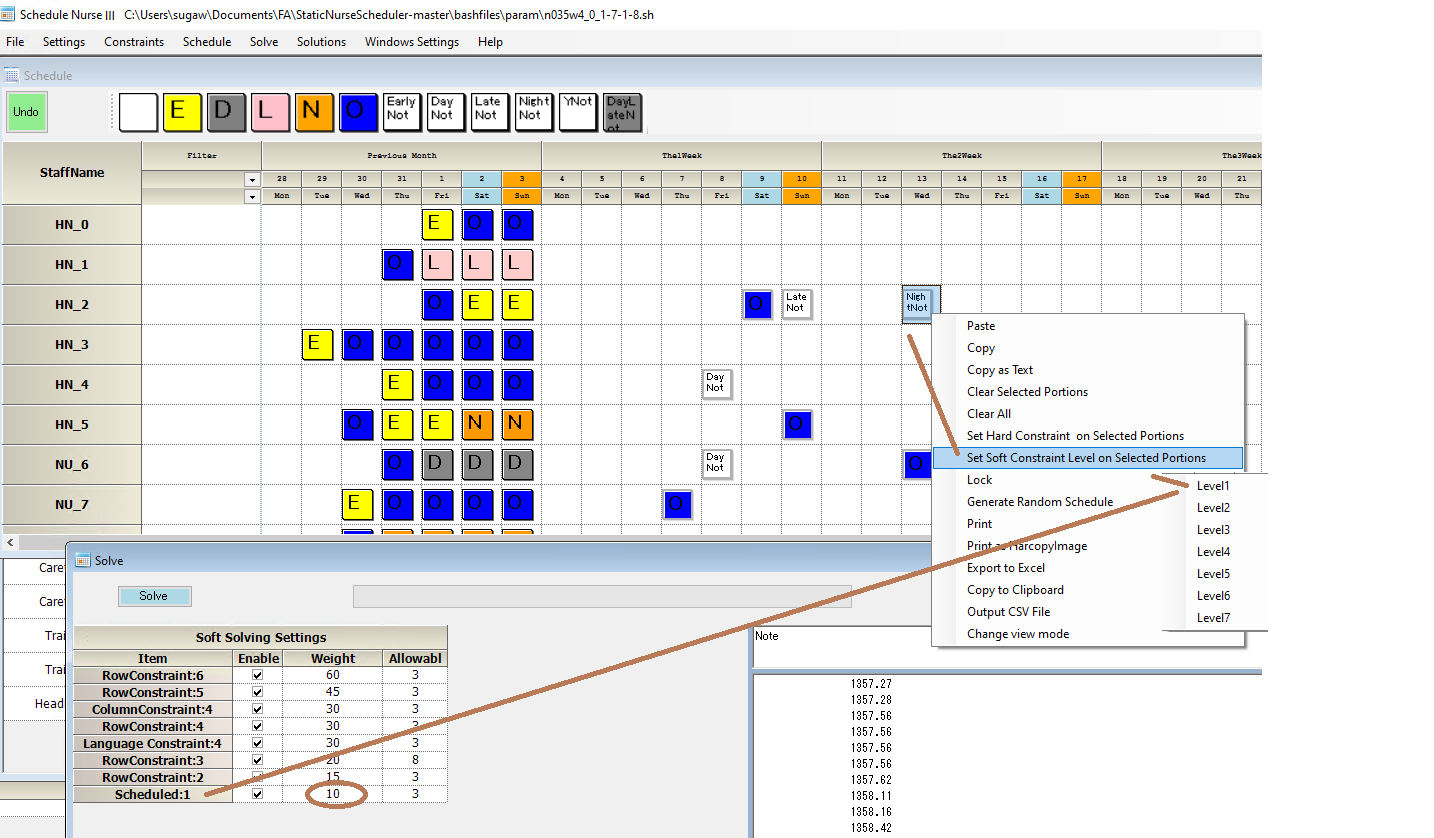### Insuﬃcient staﬃng for optimal coverage (30):

The number of nurses for each shift for each skill must be equal to the optimal requirement. Each missing nurse is penalized according to the weight provided. Extra nurses above the optimal value are not considered in the cost.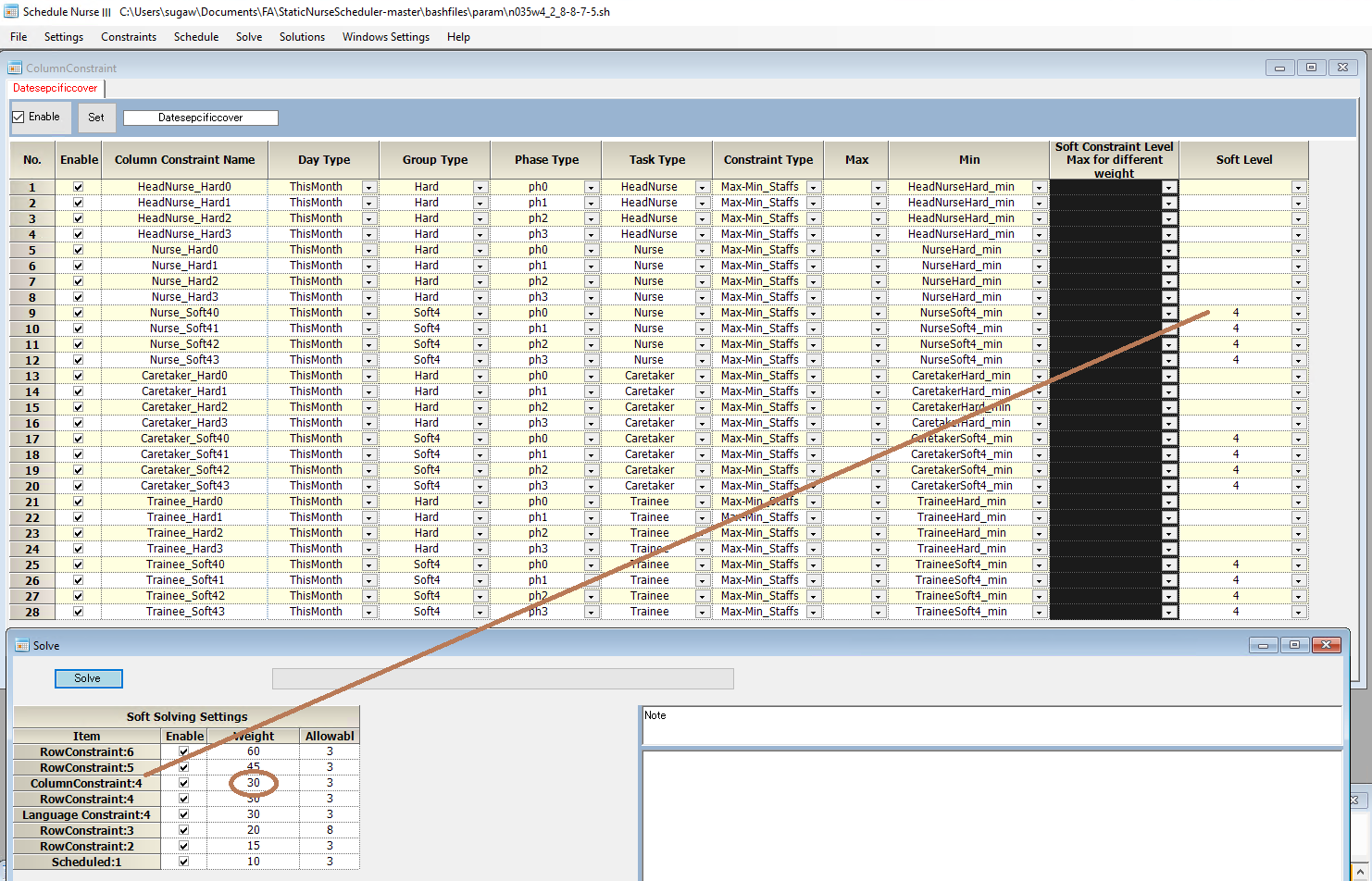The column constraints above refer to the following cardinal’s table as a min value vector.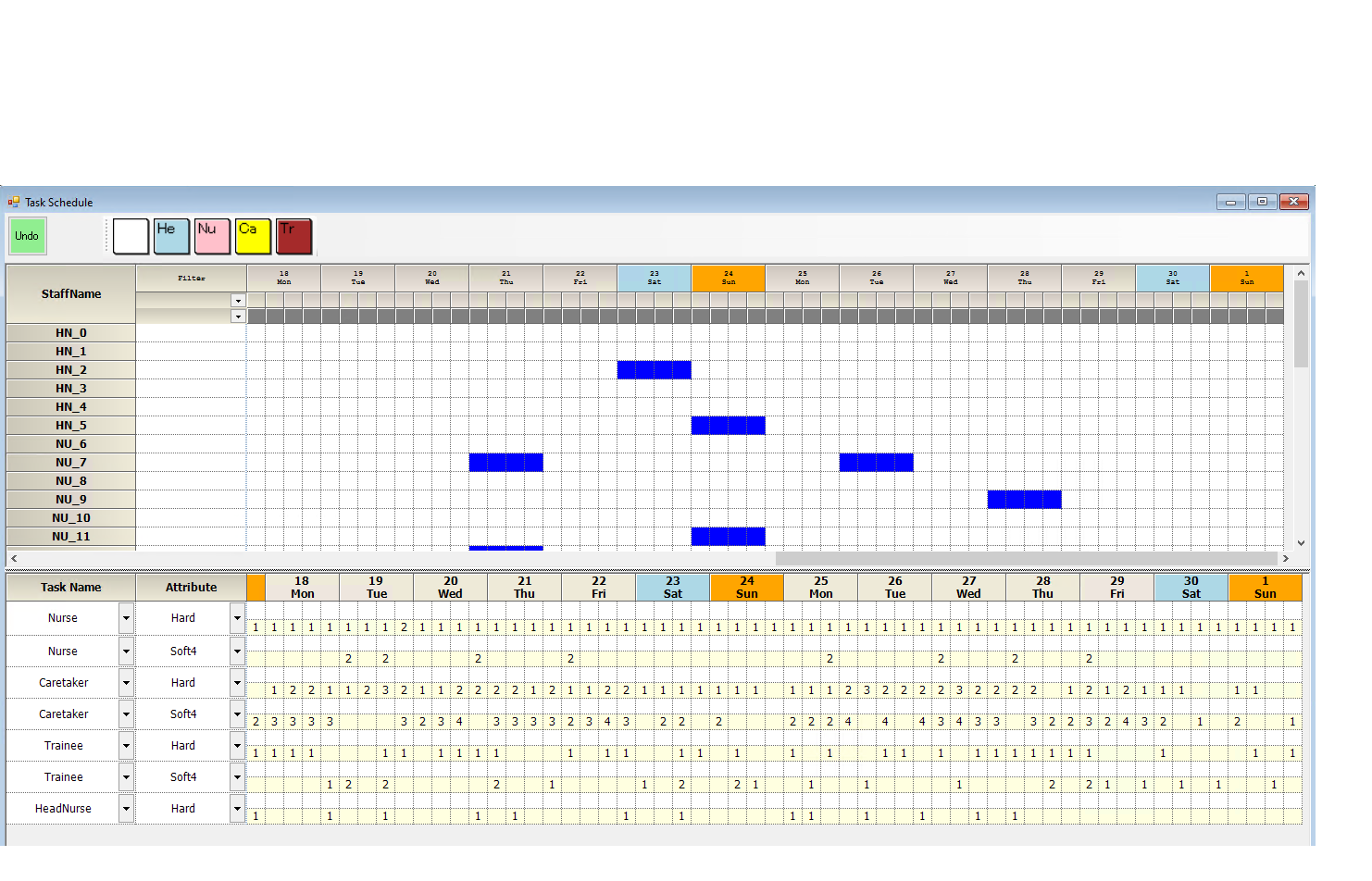### Total assignments (20):

For each nurse, the total number of assignments (working days) must be included within limits (minimum and maximum) enforced by their contract. The diﬀerence (in either direction), multiplied by its weight, is added to the objective function.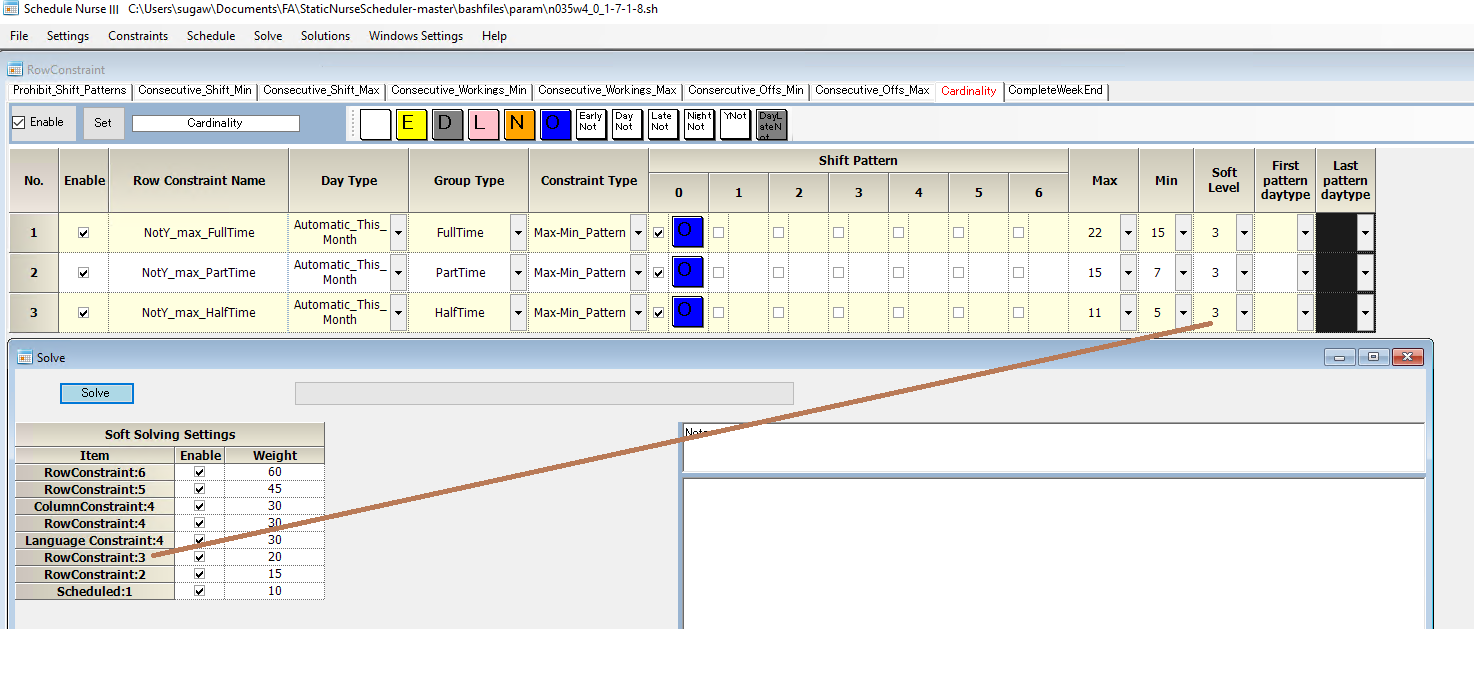You can calculate the total cost with the offset by a linear function.
For example, cardinality 5-11 has no cost in HalfTime Group. Cardinality of 12 has cost 20. 13 has cost 40, and 14 has cost 60.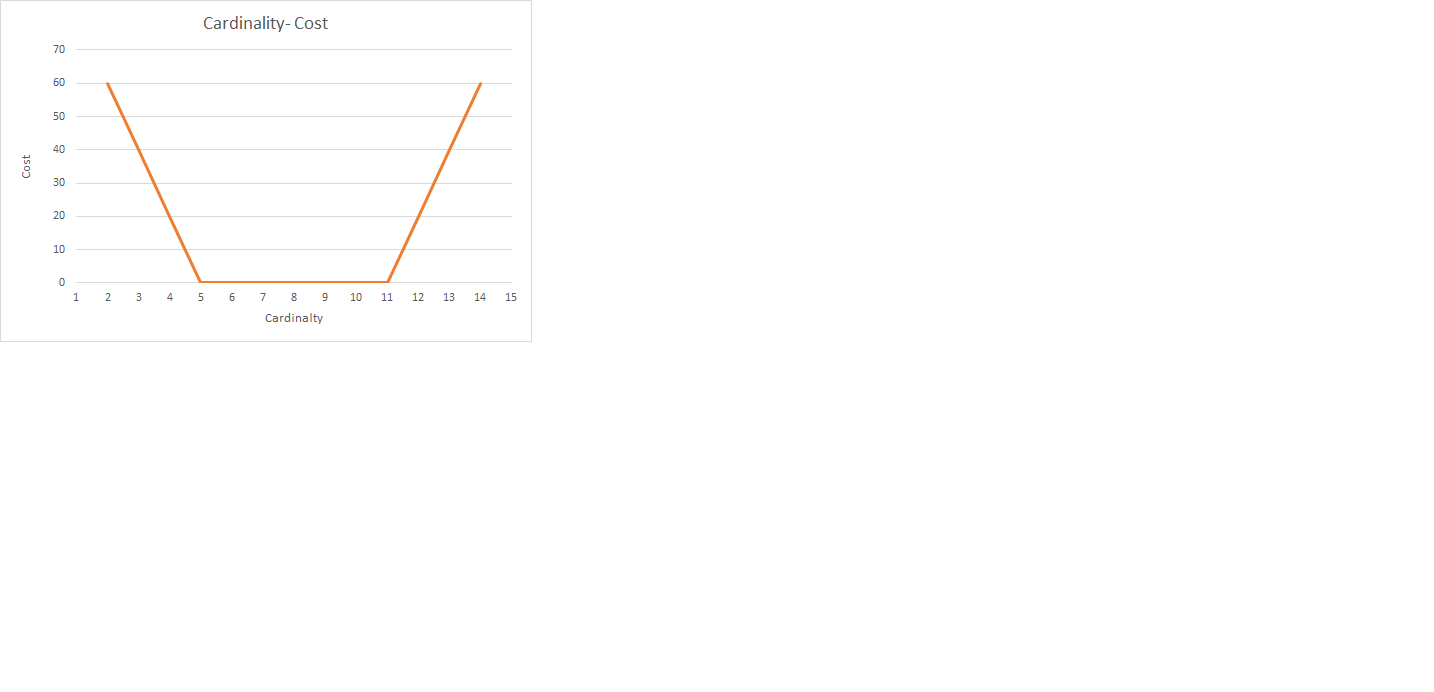### Consecutive assignments (15/30):

Minimum and maximum number of consecutive assignments, per shift or global, should be respected. Their evaluation involves also the border data. Each extra or miss- ing day is multiplied by the corresponding weight. The weights for consecutive shift constraint and for consecutive working days are respectively 15 and 30.

The table below NightShift has spec. 4 of the min-consecutive shift. So we penalize a weight for three consecutive patterns, two weights for two consecutive ways, and three weights for a single Nightshift.## What is ✓ in a row constraint pattern?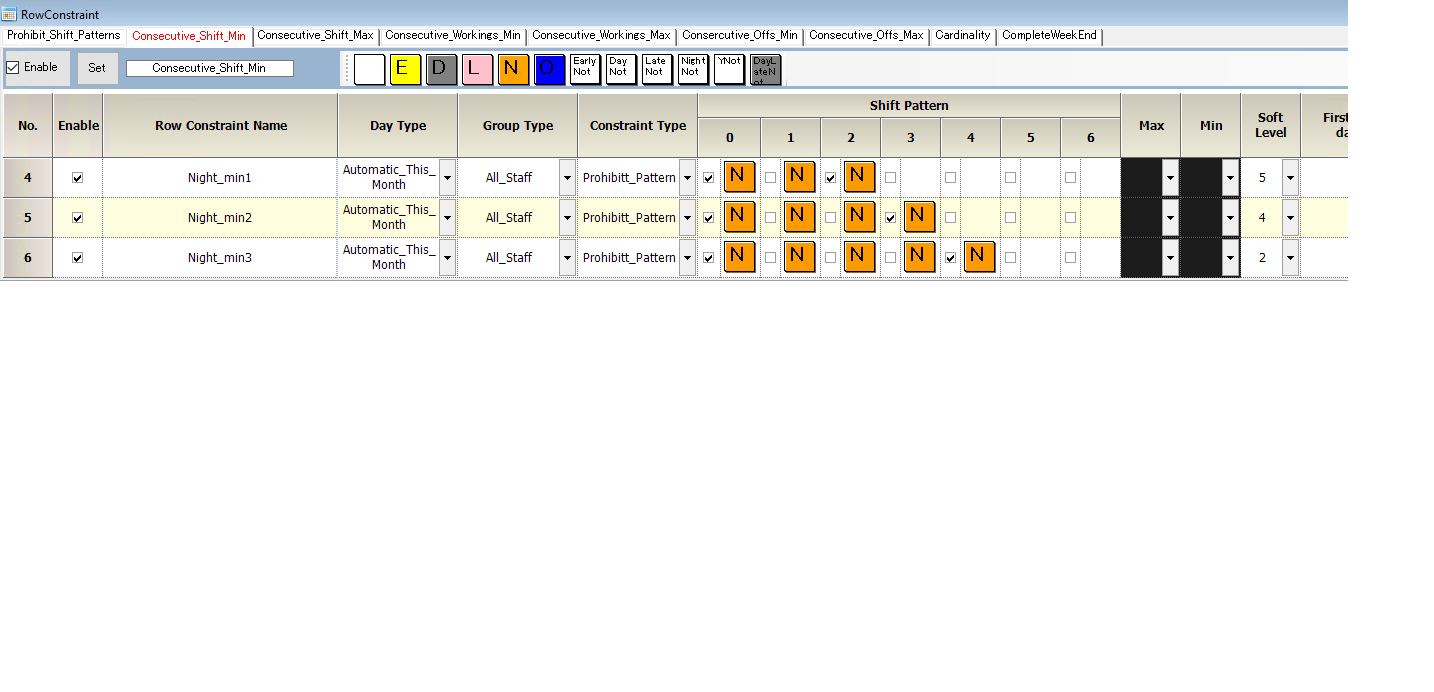It is a complement set for the shift.
So, ✓N means Day Shift or Early, or Late, or Y in the set of shift definitions below.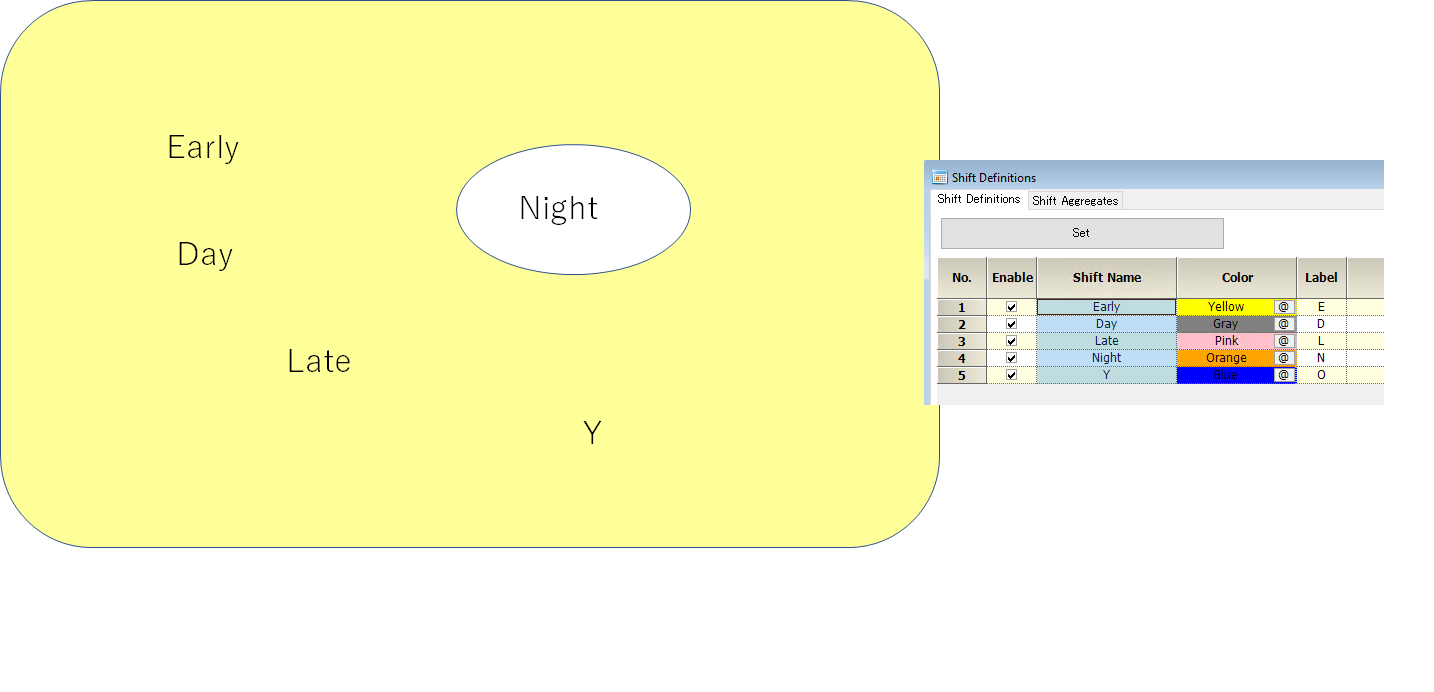## How do you write the ABCD pattern?

Use Prohibit-constraint in a row constraint.
Let us assume that A, B, C, and D are Sets that do not intersect each other . We first focus on A and B. The first constraint is that the pattern of A’s complement followed by B is not allowed.
The second constraint is A followed by B’s complement is not permitted.
In the same way, we can write the rules for C and D.We show the result in the table below.
Note this is an example of a solution. Solutions are almost infinite if we use only the constraints above.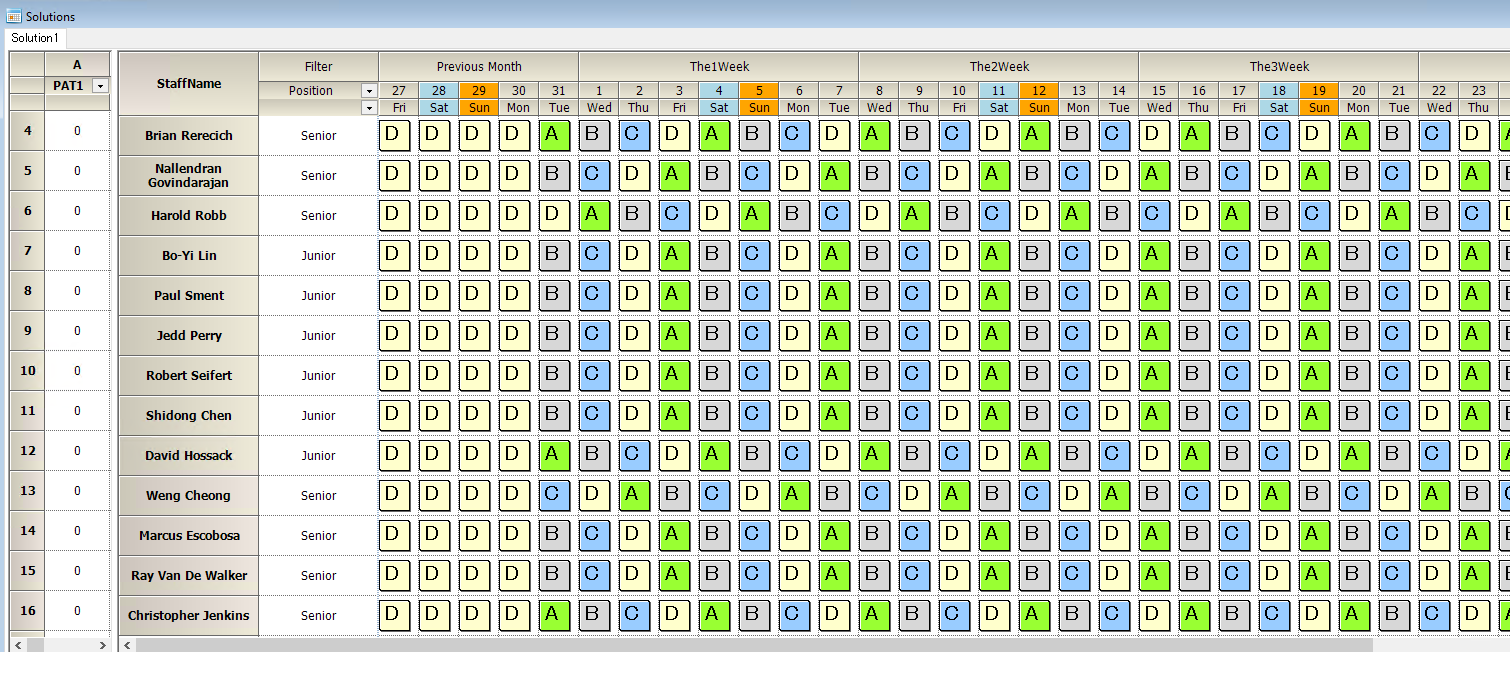## How do you describe a maximum consecutive pattern?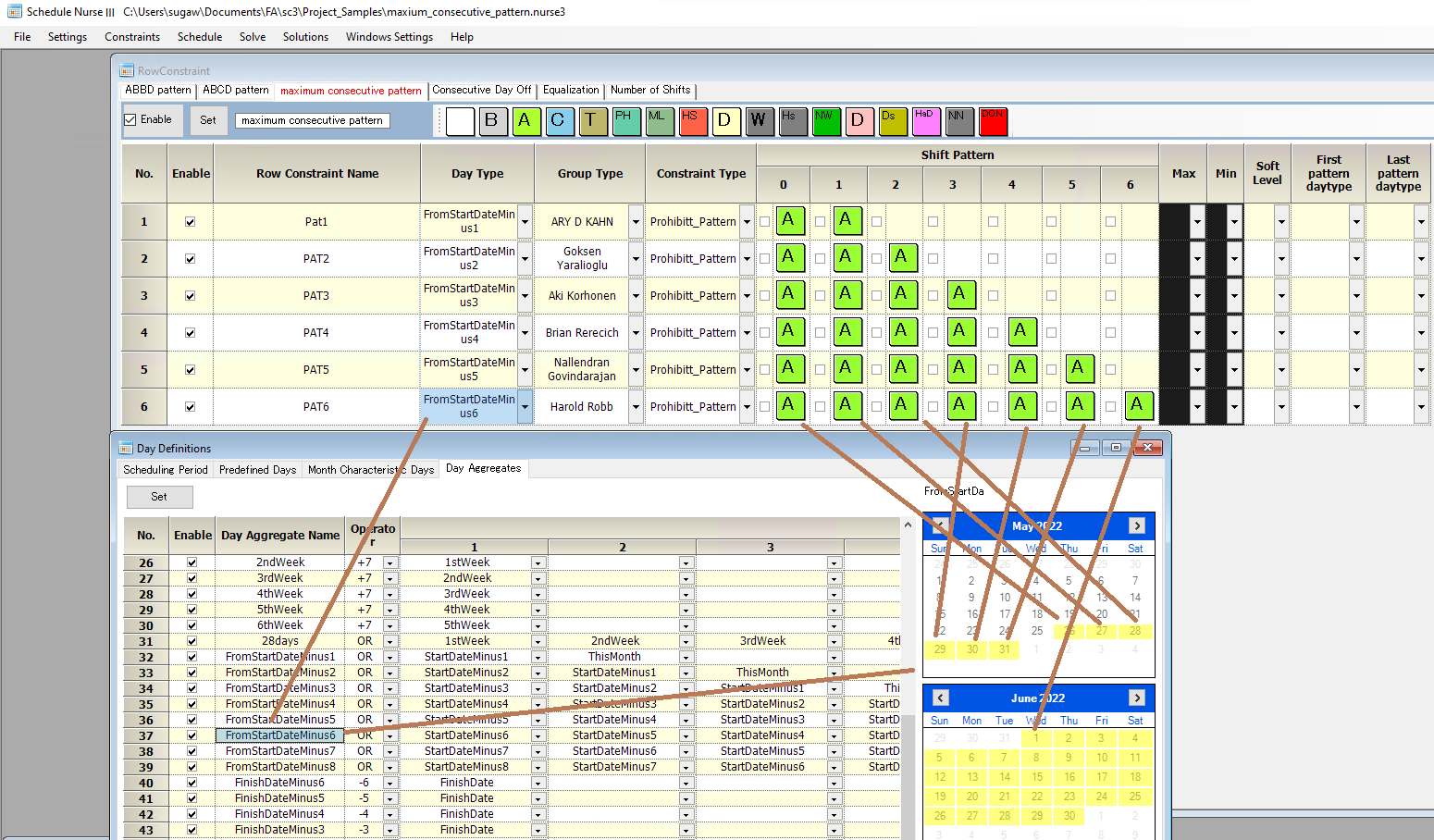Note that the DayType must be changed according to the pattern length as shown below to activate the constraint from the last month continuously.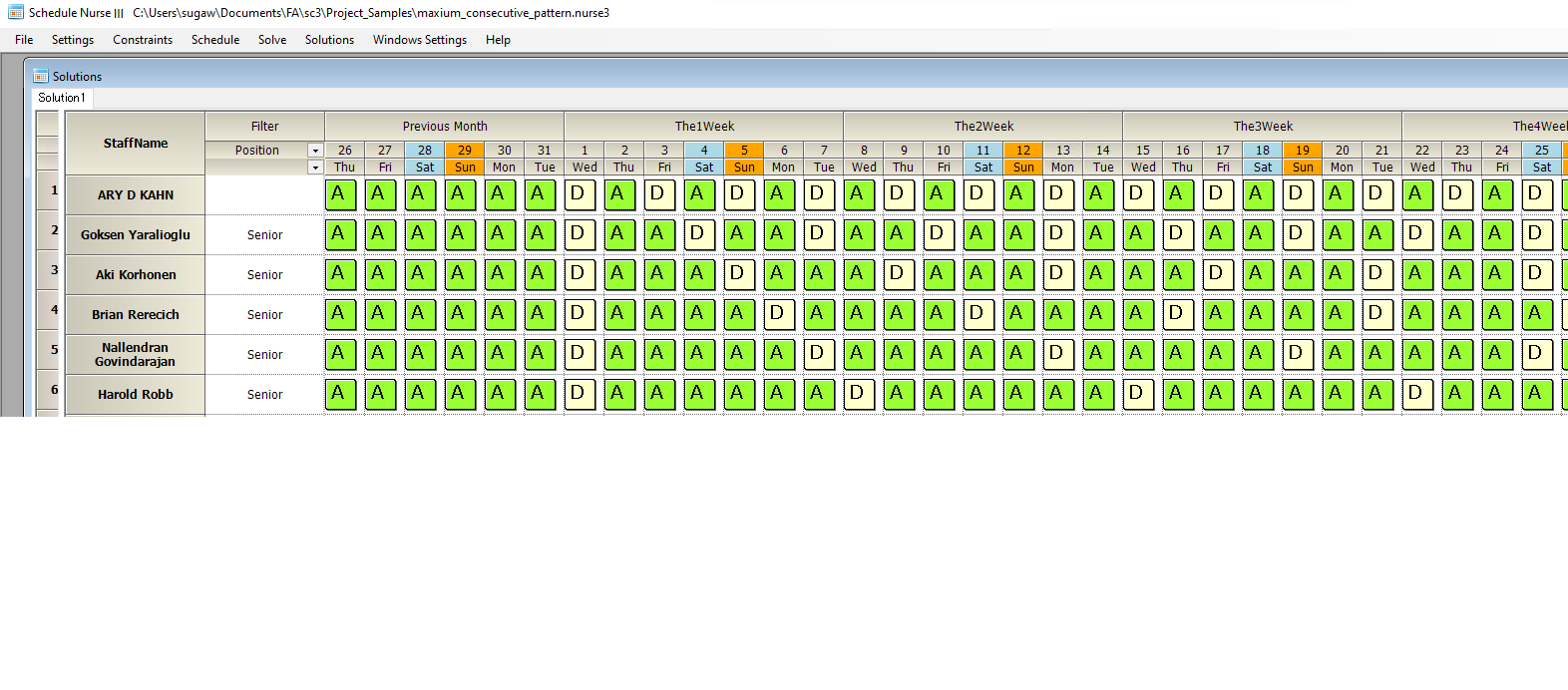AutomaticThisMonth will automatically replace the DayType with the one determined by the pattern length.## How do you describe a minimum consecutive pattern?

It needs several constraints to do this.
For example, if you need a minimum of three consecutive A shifts, you must prohibit two consecutive A shifts and a single A shift.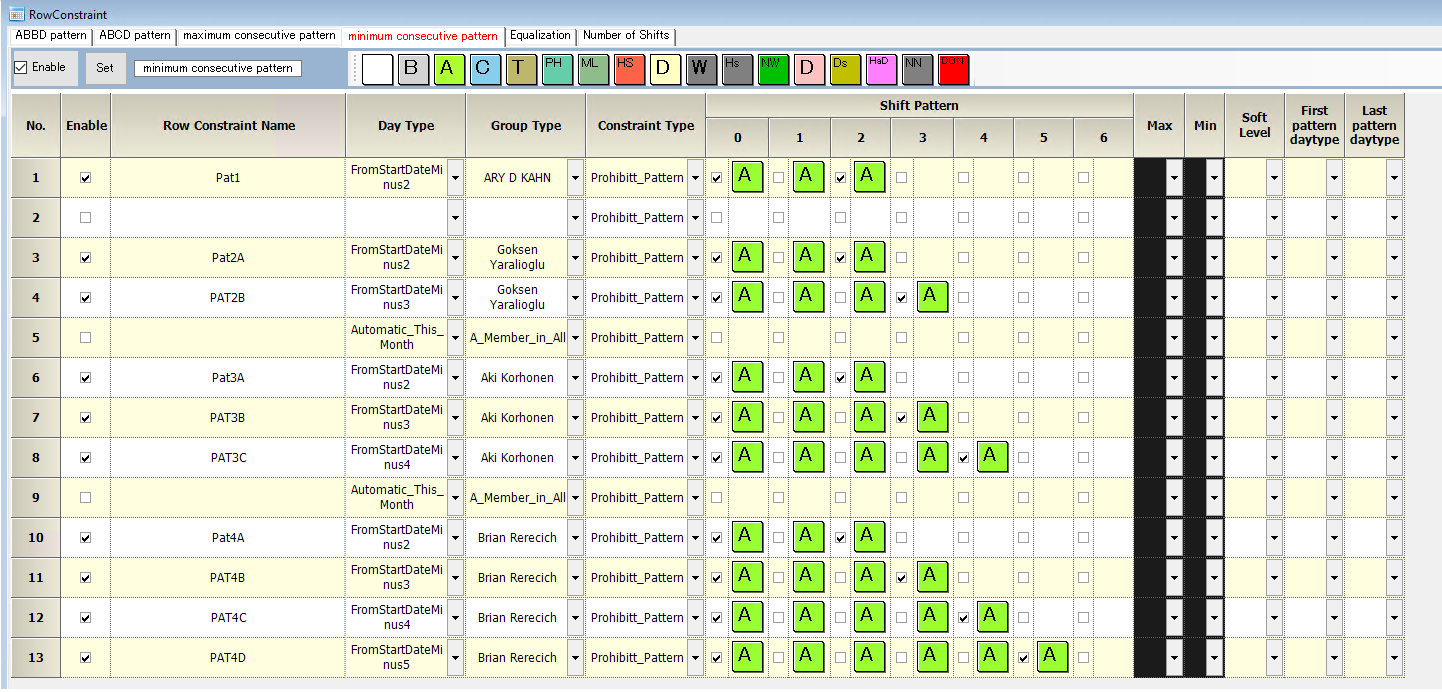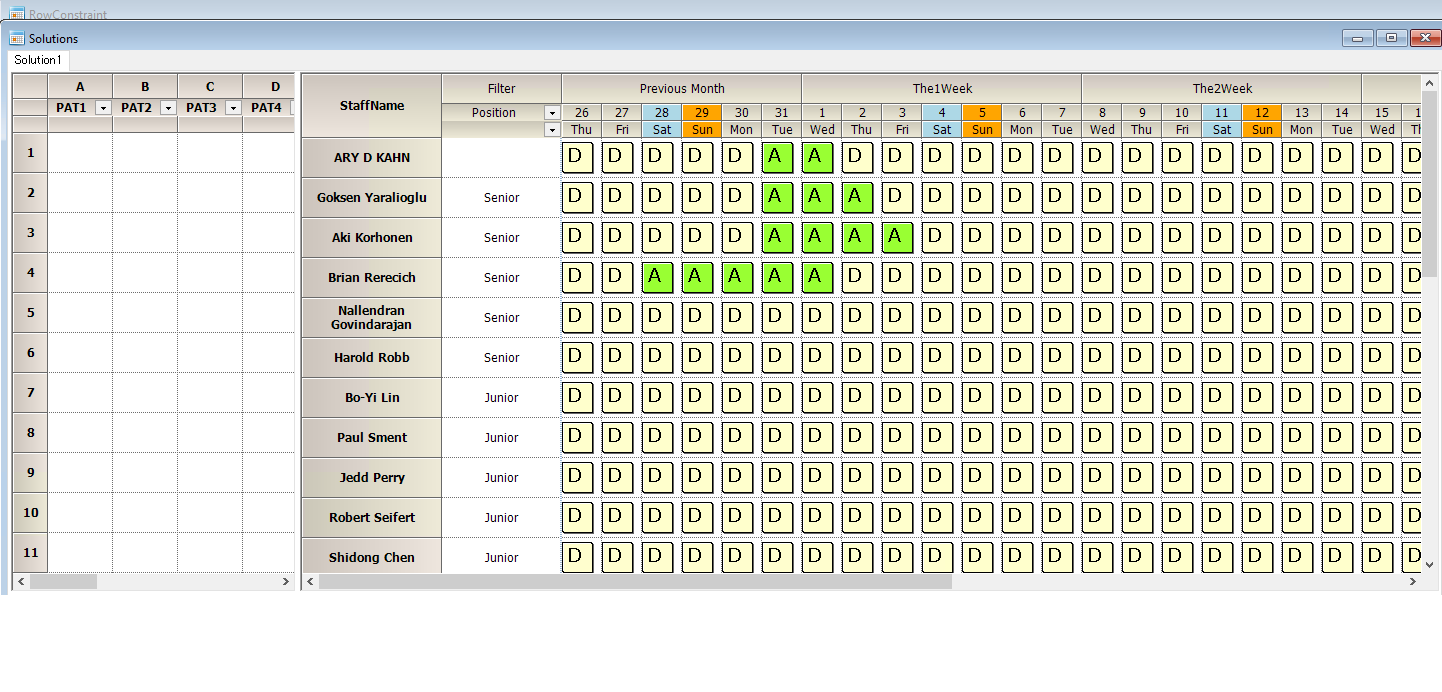## How do you describe an ABBCD pattern?

Writing down the constraint is not so simple because of the BB pattern.
Consider the BB pattern as a single symbol b. Then we only need to describe the AbCD pattern.　 Now, we can write constraints as follows.
A→B
A←b
b→C
B←C
C→D
C←D
Finally, b requires a minimum of two consecutive Bs.
~B & B & ~B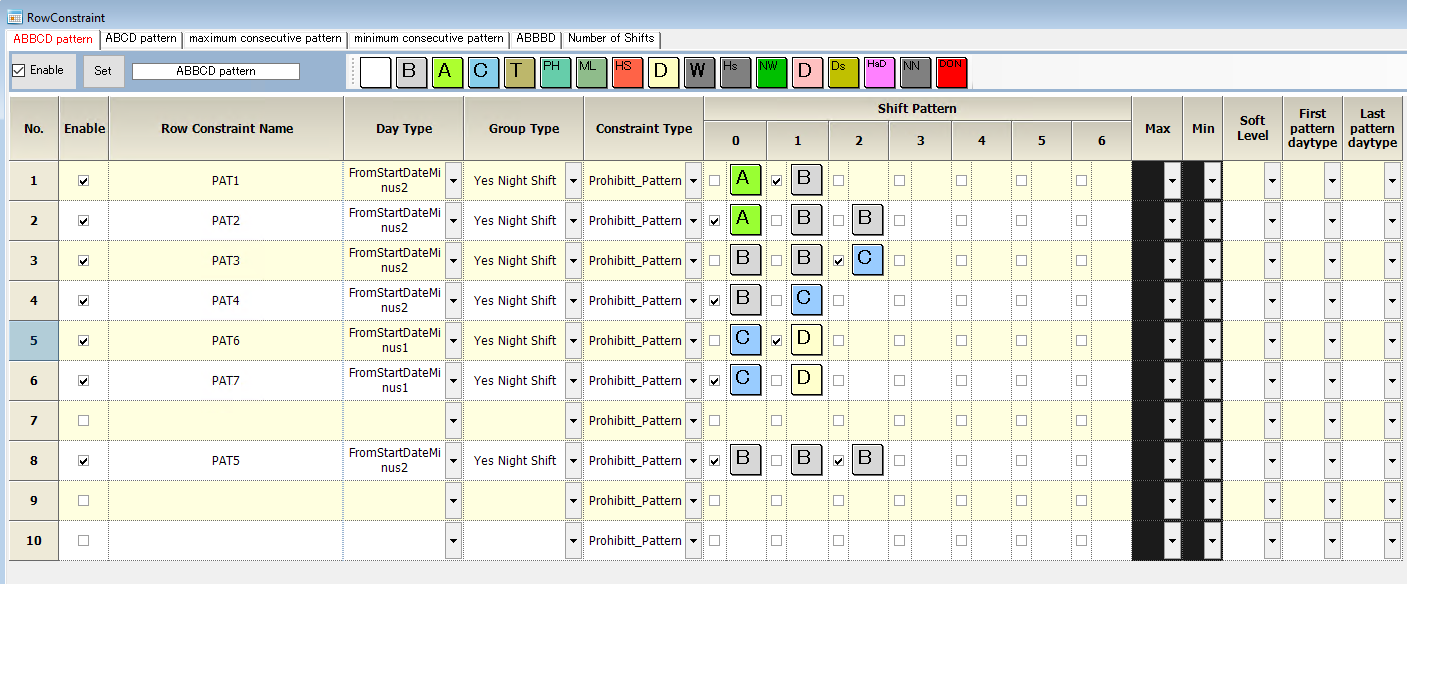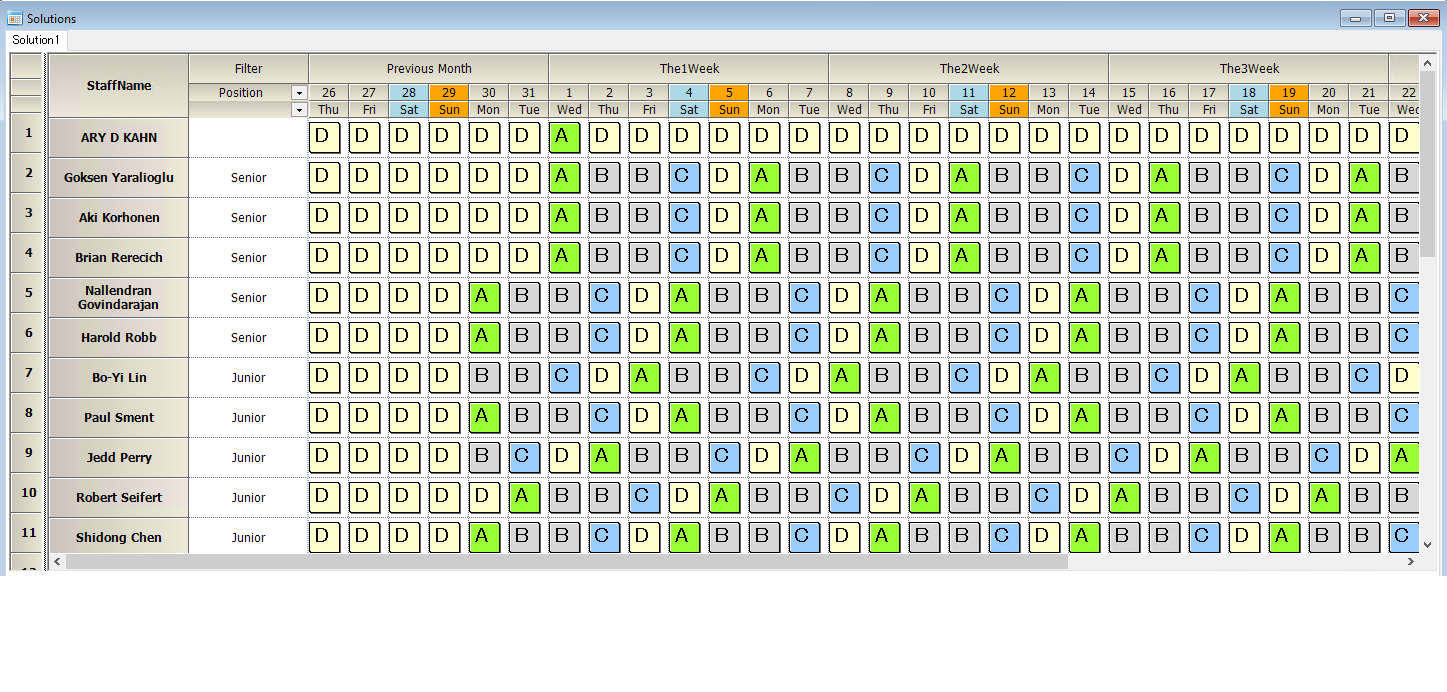## How do you describe an ABBDCD pattern?

Writing down the constraint is not so simple because of the BBB pattern.
Consider the BBB pattern as a single symbol b. Then we only need to describe the AbCD pattern.　 Now, we can write constraints as follows.
A→B
A←b
b→C
B←C
C→D
C←D
Finally, b requires a minimum of three consecutive Bs.
~B & B & ~B
~B & B & B ~B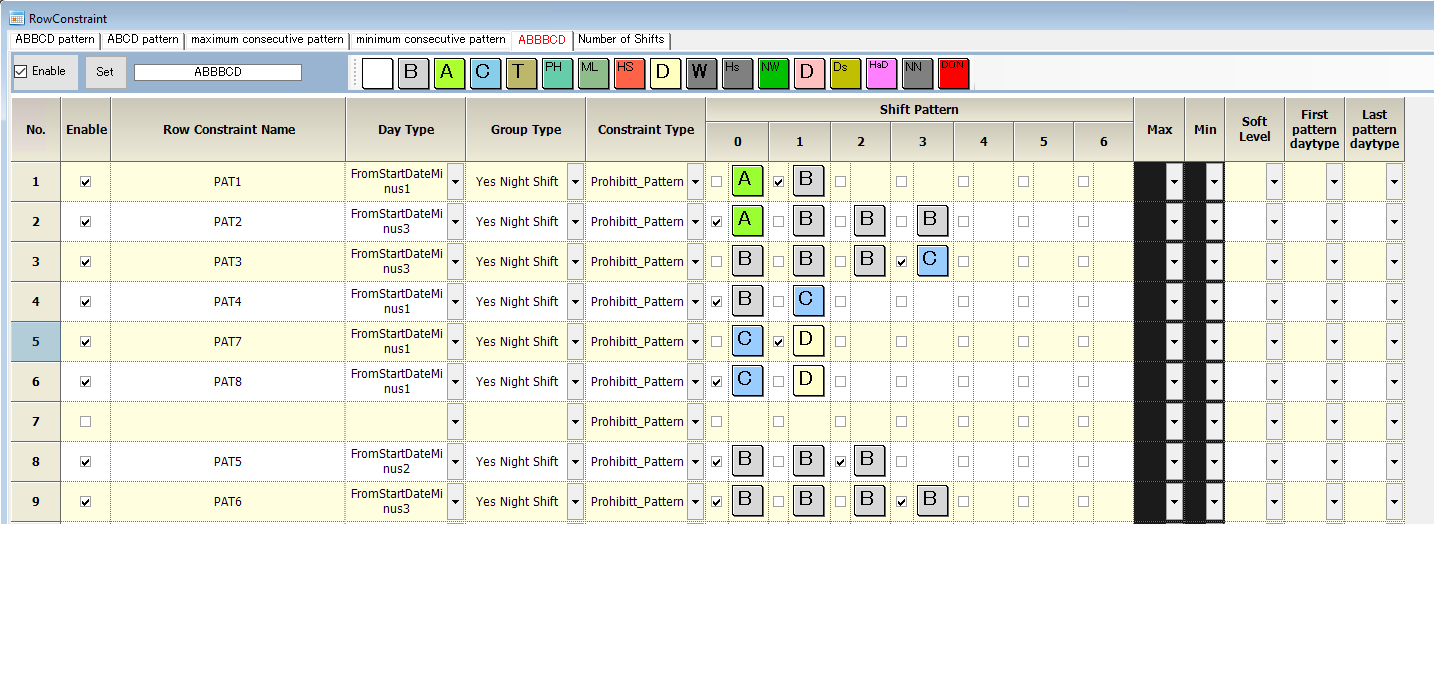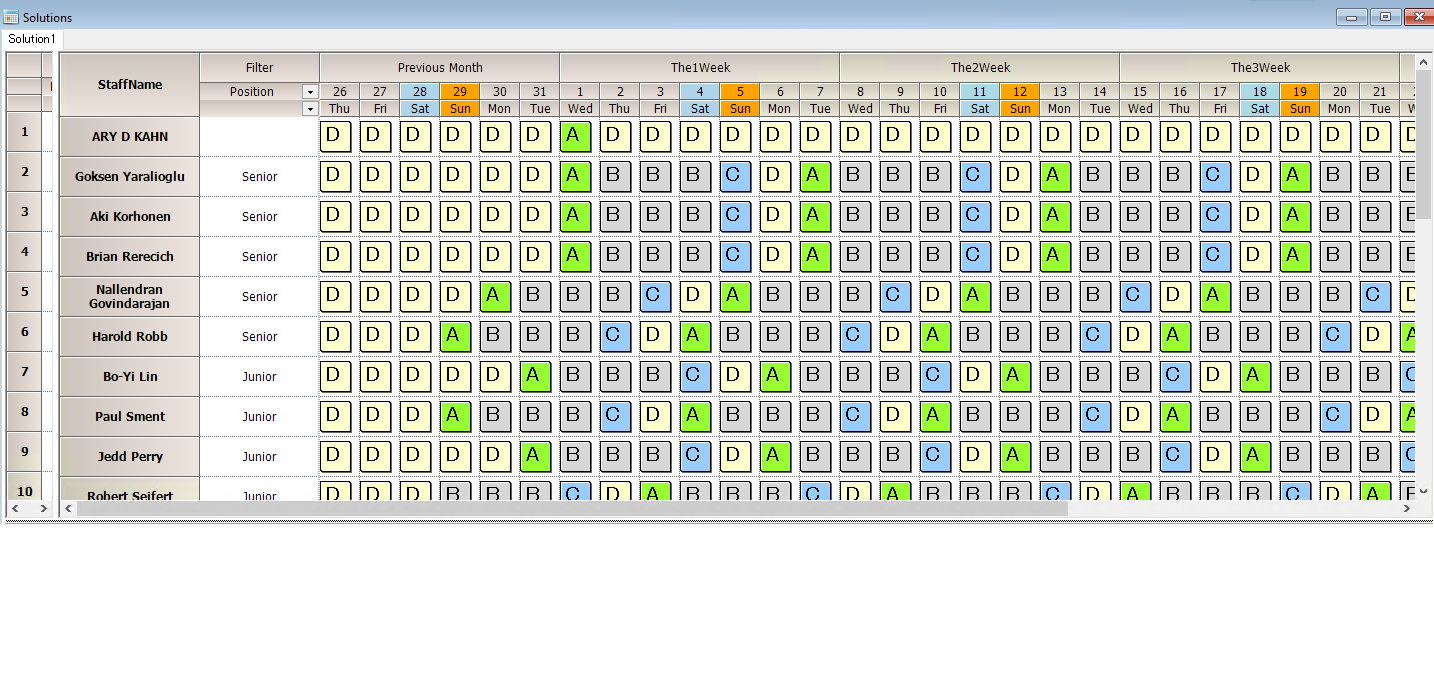## Can I define shift types that the solvers won’t assign?

Yes, You can define the shift-type by unchecking Automatic Shift.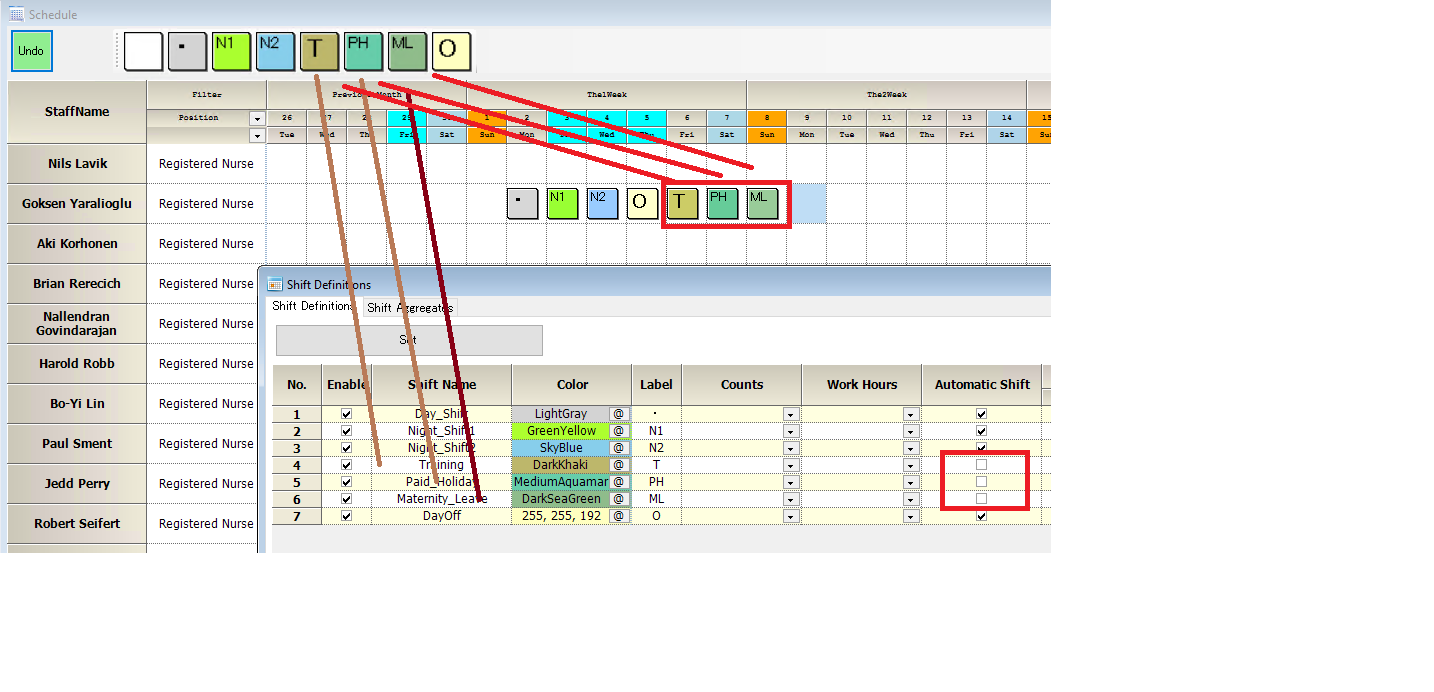Note only a scheduled view assigns Training, Paid_Holiday, and Maternity_Leave, not by the solver.## How do I model “both Saturday and Sunday on or off” constraints?

You can write it by the figure below. (“O” is day-off in this example)
For example, use Sat on the first day of the pattern.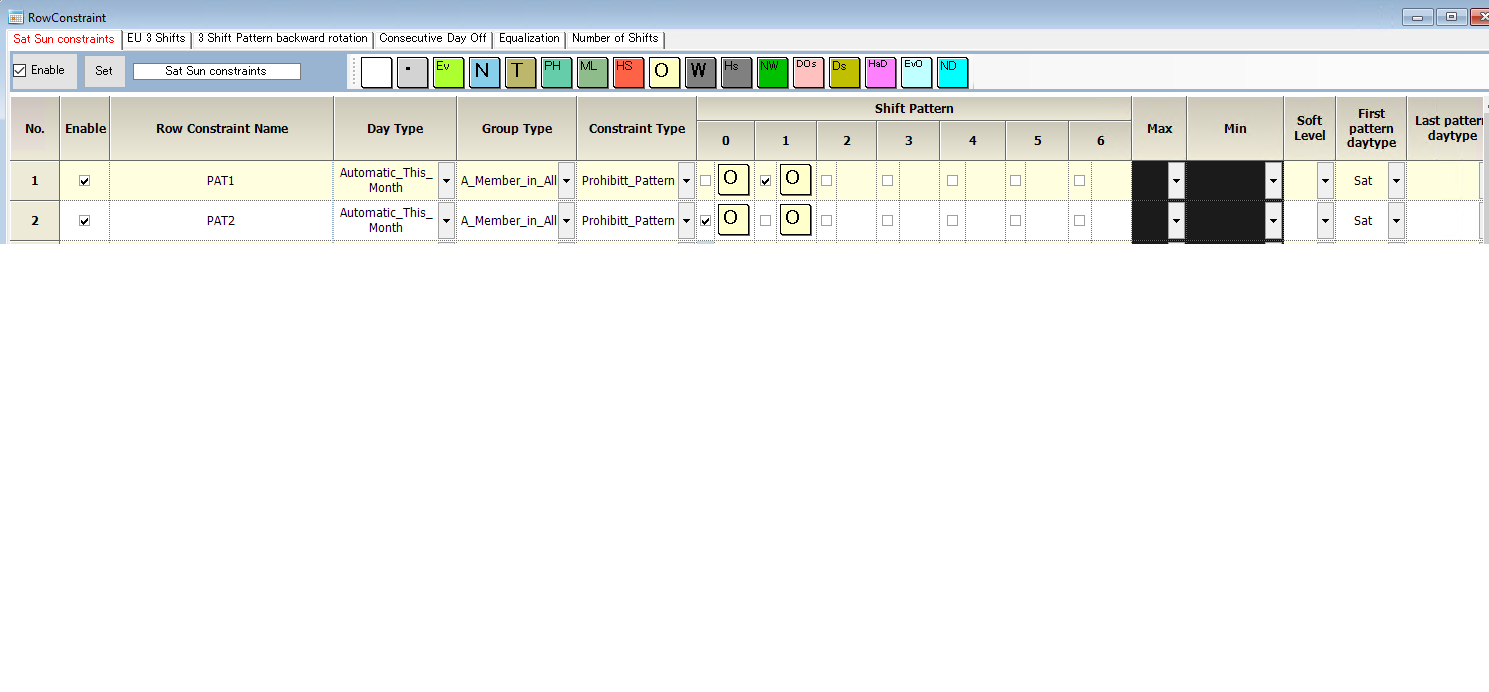## How do I model “no night shift before a weekend off” constraints?

You can write it by the figure below. (“O” is day-off ,and “N” is night-shift in this example)
For example, use Fri on the first day of the pattern.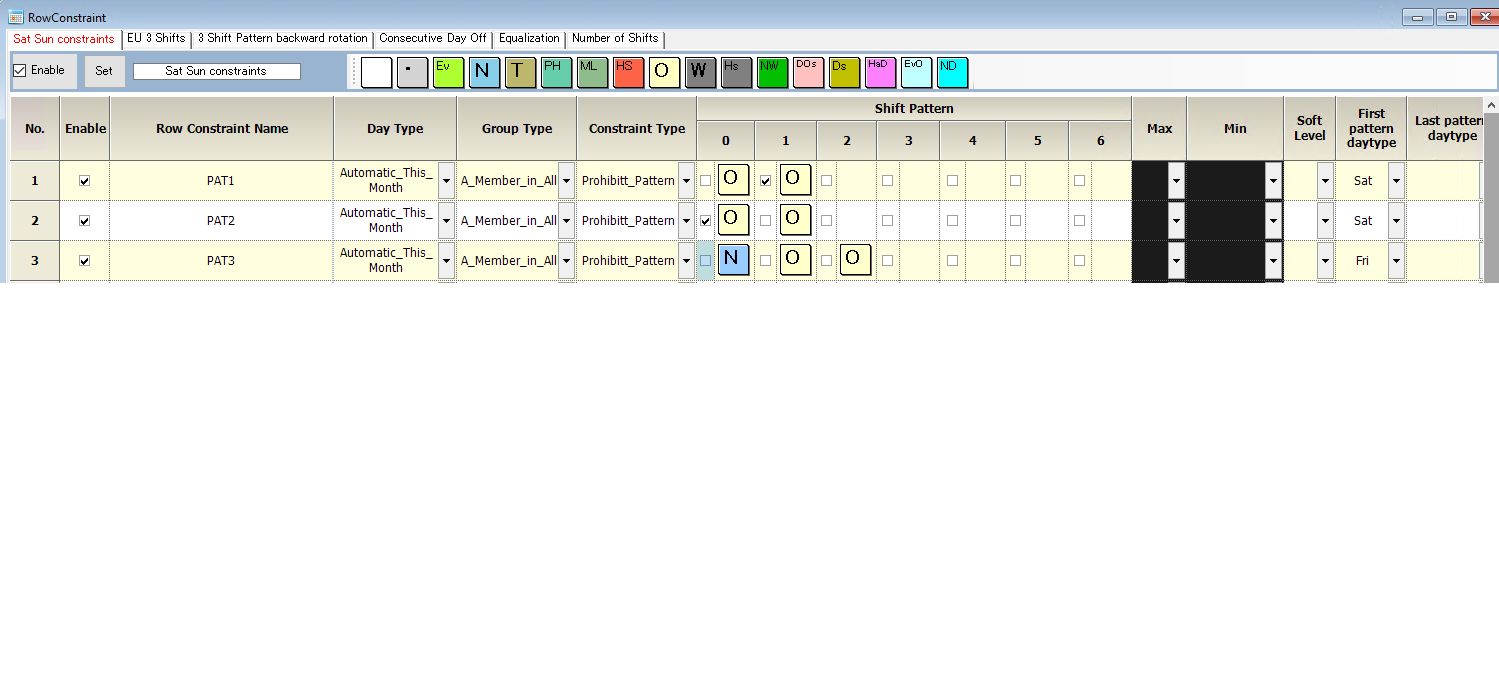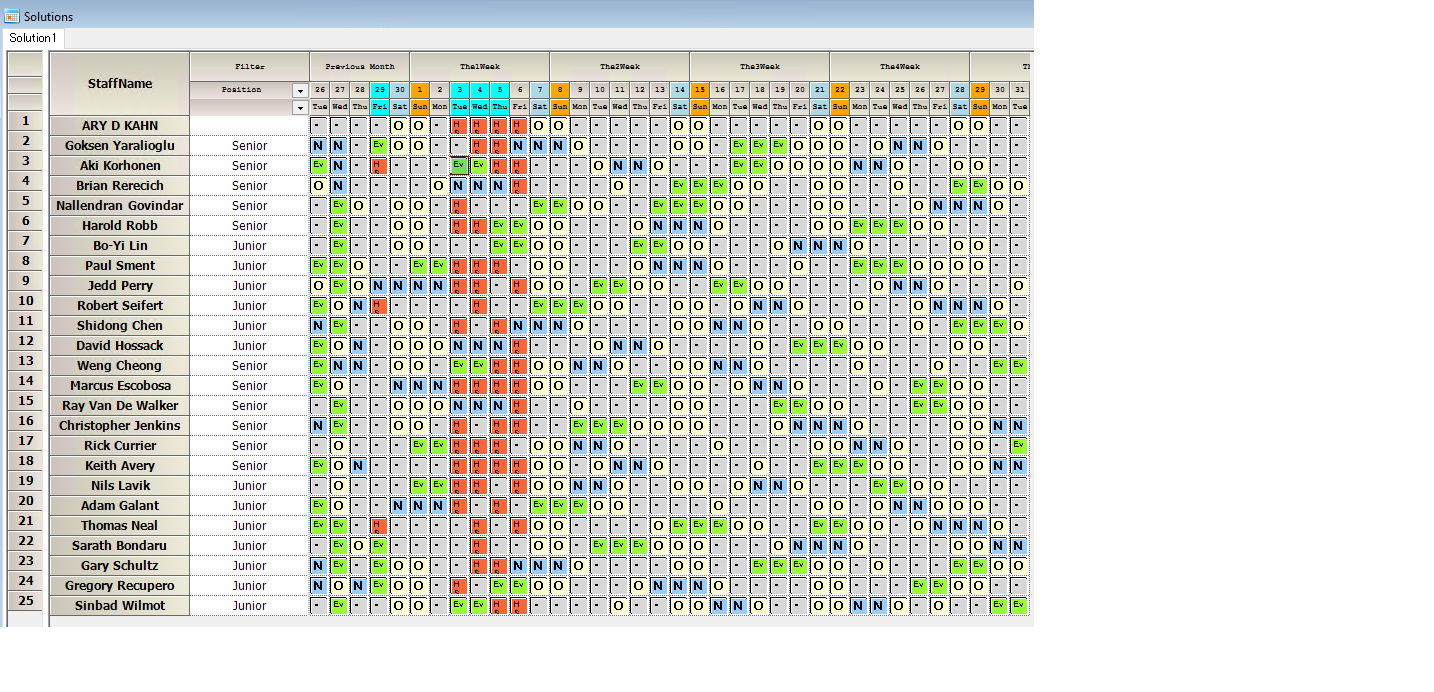## Algorithm 1 and 3 are selectable. Which one should I use?

A. There is a clear difference in purpose and application.

### When designing constraints

Please use Algorithm 1. In designing constraints, as a debugging tool, root cause analysis is essential when there is no solution. Note that only Algorithm1 is effective for root cause analysis.

### Normal use

In most cases, Algorithm1 is the fastest and most stable.

### Number of CPUs

The number of CPUs can be one; 2 or more CPUs are also possible, but it does not make much difference.

### For exact solutions

Algorithm1 outputs Near-Optimal solution in principle. If the objective function value (UB) is 0, it is Optimal. If it is output after a timeout period and UB is not 0, it may not be an exact solution. In such cases, if you want to find the exact solution (physical limit value), then Algorithm 2, 3, or 4 are the choices. Official support is only for 1 and 3, but 2 and 4 will also work if configured.

The Academic Benchmark uses the time to reach this exact solution as an indicator, and all benchmark data for Schedule Nurse III uses Algorithm 3. There is no timeout, and it keeps running until it finds the exact solution. Therefore, it never ends up with a worse value than Algorithm1. Also, comparing the output values of Algorithm 1 and Algorithm 3, we can see that Algorithm 1 outputs the objective function value of the exact solution or a value very close to it. (To stop the algorithm in the middle of the solution, press the Abort button.)

#### Algorithm 3

1. Recommended environment A modern processor with at least 32 GB of memory and a CPU with at least 8 cores. (Schedule Nurse III uses full CPU power with full threading.)

2. Although it can be used with almost all instances, please make sure that it works with Algorithm 1 before use.

3. Situations in which Algorithm 3 shows its power

Algorithm 3 is effective when Algorithm 1 is time-consuming cases (especially when there are many hard schedules and soft constraints). It is also useful when you want to know the LB value.

#### Algorithm 2

We solve the problem using HiGHS, an open source MIP solver. For small instances (about 10 staff members), it may work well. However, for medium or larger huge instances, it will take longer and will likely not yield a solution.

#### Algorithm 4

Algorithm 3 is a fusion of Algorithm 4 and Algorithm 1. Algorithm 1 is executed by instances, but Algorithm 4 does not have this phase.

## How do I move on next month with continuity?

We explain using the project below.
Maximum-length-series-of-shifts slow down solving speed

### Change Scheduling Horizon

The following fig. shows the current month, Jul.1.2022 - Jul.31.2022.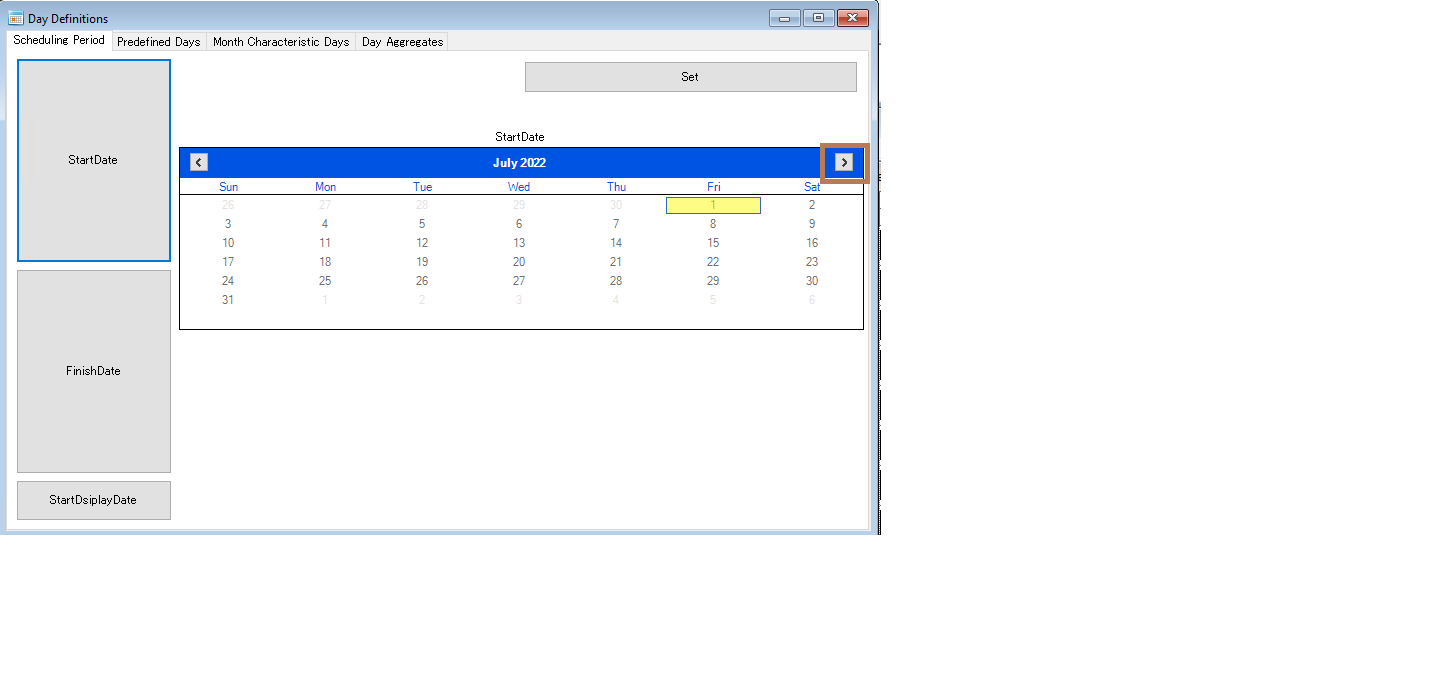We move Aug.1.2022 -Aug.30.2022.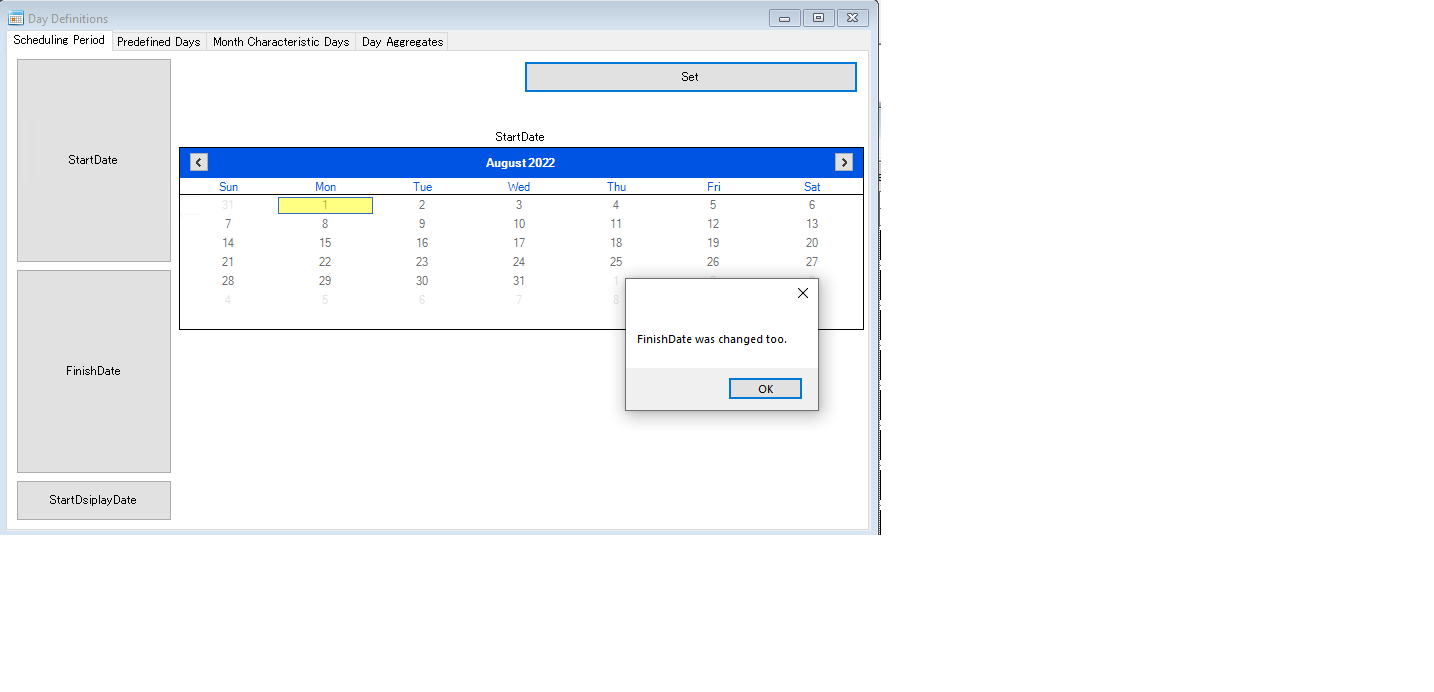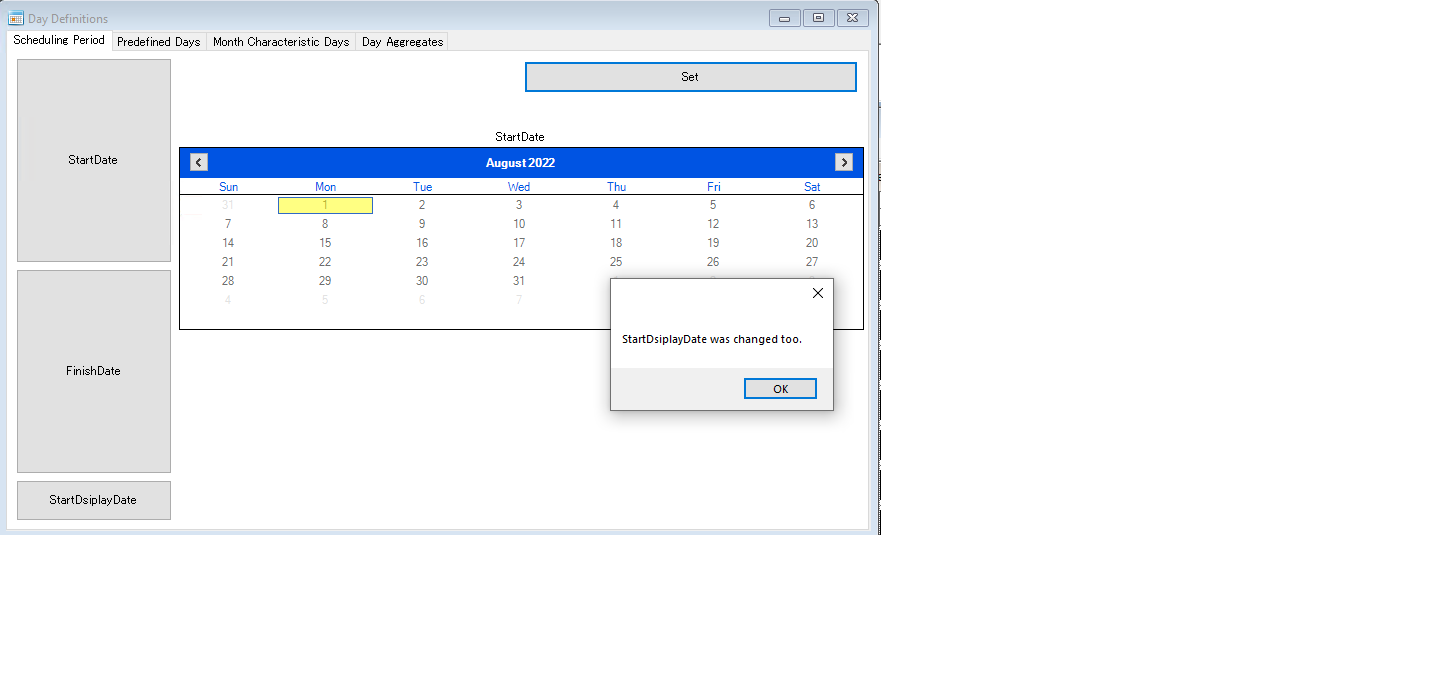Make sure we are in Aug.1.2022-Aug.30.2022.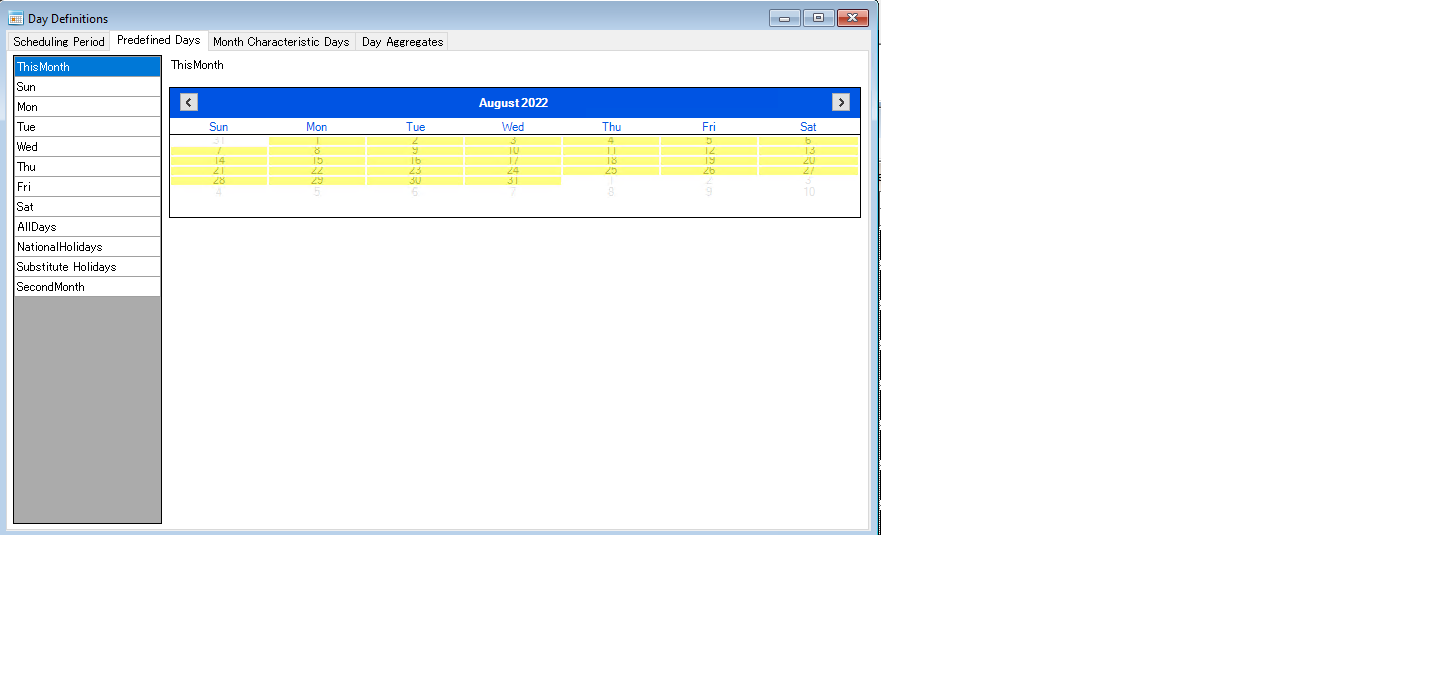### Send the current month solution to Scheduling Input View

Right Click on Solution View. Click “Send to Input View”.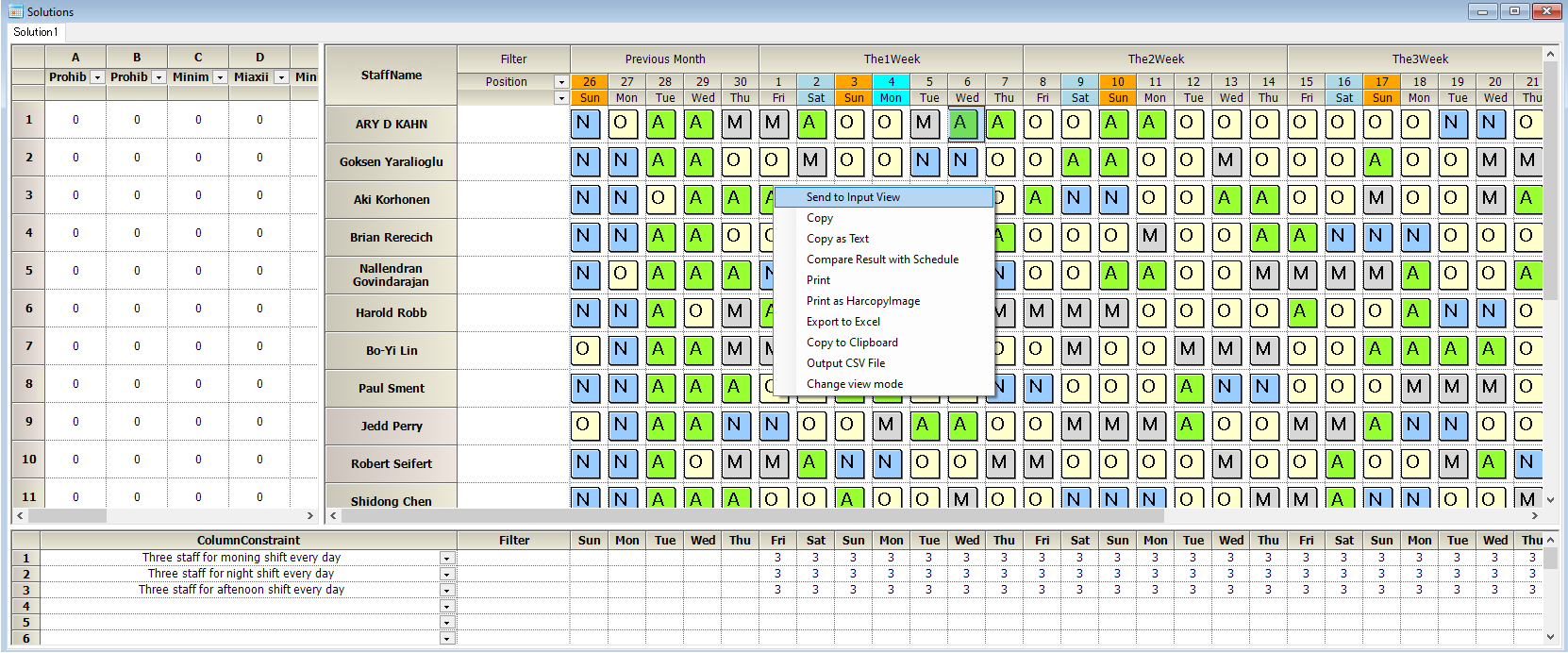Note your data(the last month solution) are located in "Previous Month"on Shift Scheduling View. Input schedules in this month.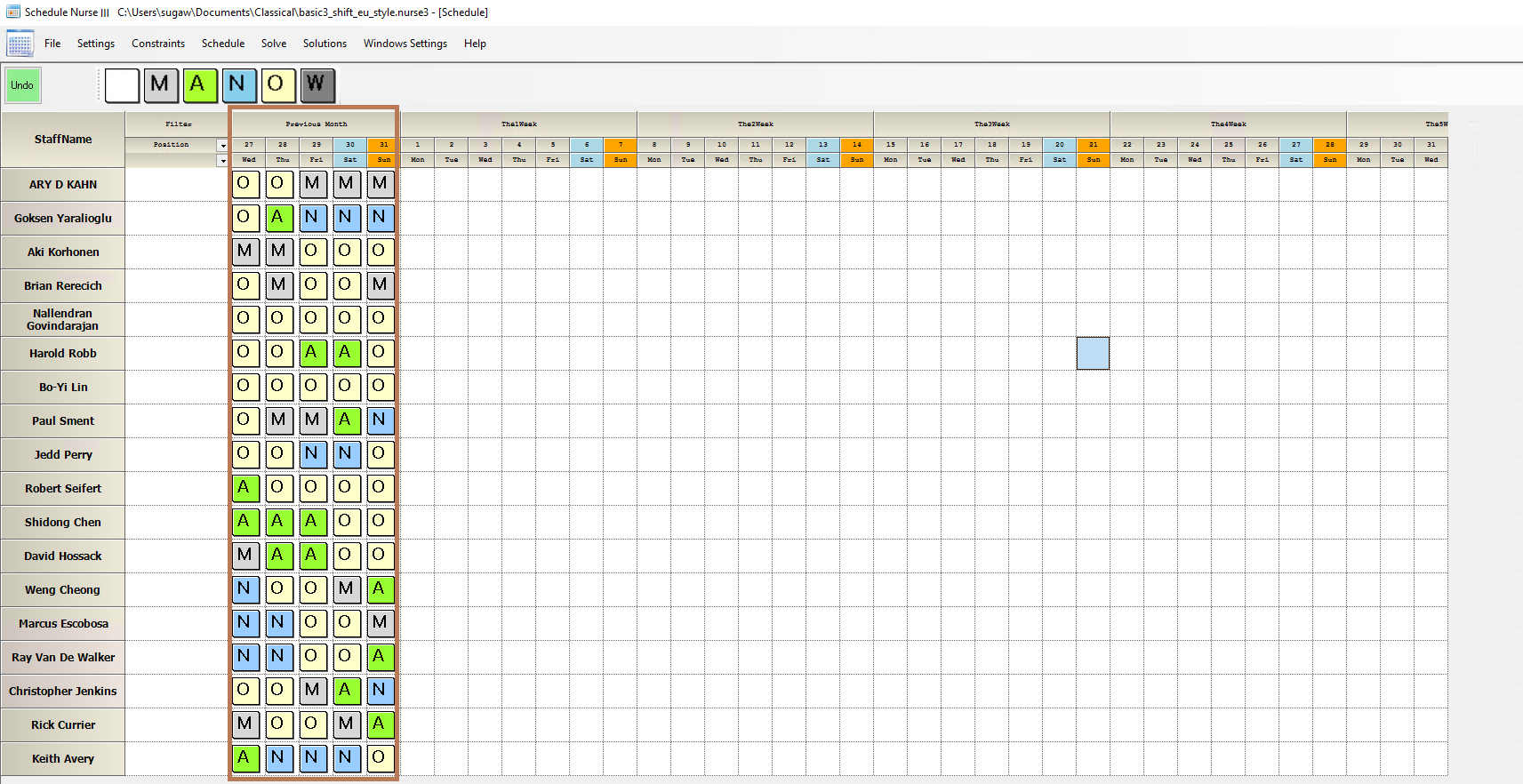Note your constraint requires 5 days data in "Previous Month" for continuity.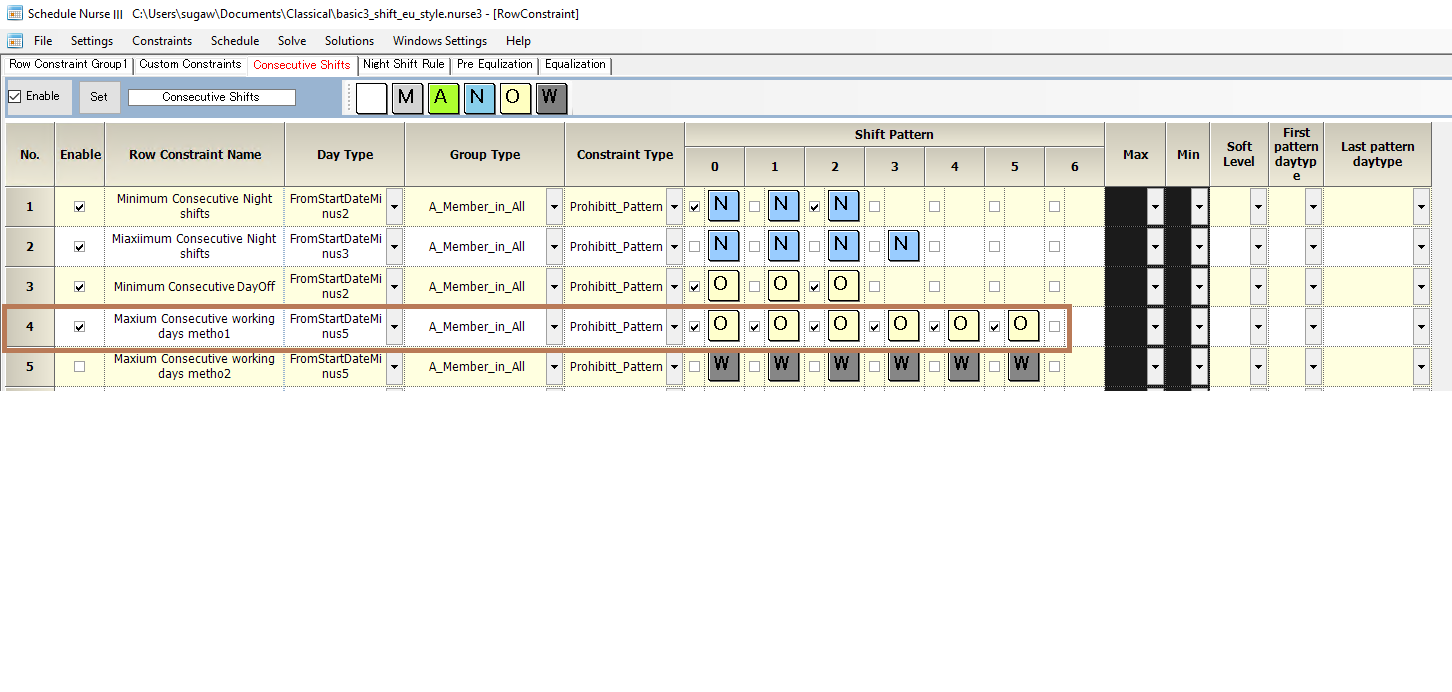### Get Solution this month

Normally, you can get your solution by just solving button,i.e. No need to modify your constraints.Make sure continuity.